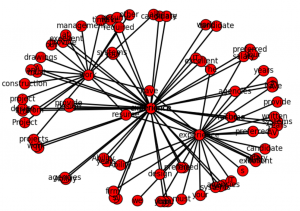# Data Visualization of Word Correlations with NetworkX

This is a continuation of my previous post, found here Combining Machine Learning and Data Scraping. Data visualization is added to show correlations between words. The graph was built using NetworkX python library.
The input for the graph is the array corr_data with 3 columns : pair of words and correlation between them. This was calculated in the previous post.

In this post are added two functions:
build_graph_for_all – it is taking words from matrix for the first N rows and adding to the graph.
The graph is shown below.The Second function build_graph is taking specific word and adding to graph only edge that have this word. The process is repeating but now it is adding edges to other words on the graph. This is recursive function. Below in the python code are shown these functions.

Python computer code:

``````
import networkx as nx
import matplotlib.pyplot as plt
G=nx.Graph()

existing_edges = {}

def build_graph(w, lev):
if (lev > 5)  :
return
for z in corr_data:
ind=-1
if z == w:
ind=0
ind1=1
if z == w:
ind ==1
ind1 =0

if ind == 0 or ind == 1:
if  str(w) + "_" + str(corr_data[ind1]) not in existing_edges :

existing_edges[str(w) + "_" + str(corr_data[ind1])] = 1;

build_graph(corr_data[ind1], lev+1)

existing_nodes = {}
def build_graph_for_all():
count=0
for d in corr_data:
if (count > 40) :
return
if  d not in existing_edges :
if  d not in existing_edges :
count=count + 1

build_graph_for_all()

print (G.nodes(data=True))
plt.show()
nx.draw(G, width=2, with_labels=True)
plt.savefig("path1.png")

w="design"
build_graph(w, 0)

print (G.nodes(data=True))
plt.show()
nx.draw(G, width=2, with_labels=True)
plt.savefig("path.png")
``````

In this post we created script that can be used to draw plot of connections between the words. In the near future I am planning to apply this technique to real problem. Below is the full source code.

``````
# -*- coding: utf-8 -*-

import numpy as np
import nltk
import csv
import re
from scipy.stats.stats import pearsonr

def remove_html_tags(text):
"""Remove html tags from a string"""
clean = re.compile('<.*?>')
return re.sub(clean, '', text)

fn="C:\\Users\\Owner\\Desktop\\A\\Scrapping\\craigslist\\result-jobs-multi-pages-content.csv"

docs=[]
start=1
file_urls=[]

strtext=""
with open(fn, encoding="utf8" ) as f:
for i, row in enumerate(csv_f):
if i >=  start  :
file_urls.append (row)

strtext=strtext + replaceNotNeeded(str(stripNonAlphaNum(row)))
docs.append (str(stripNonAlphaNum(row)))

return strtext

# Given a text string, remove all non-alphanumeric
# characters (using Unicode definition of alphanumeric).

def stripNonAlphaNum(text):
import re
return re.compile(r'\W+', re.UNICODE).split(text)

def replaceNotNeeded(text):
text=text.replace("'","").replace(",","").replace ("''","").replace("'',","")
text=text.replace(" and ", " ").replace (" to ", " ").replace(" a "," ").replace(" the "," ").replace(" of "," ").replace(" in "," ").replace(" for ", " ").replace(" or ", " ")
text=text.replace(" will ", " ").replace (" on ", " ").replace(" be "," ").replace(" with "," ").replace(" is "," ").replace(" as "," ")
text=text.replace("    "," ").replace("   "," ").replace("  "," ")
return text

print (txt)

tokens = nltk.wordpunct_tokenize(str(txt))

my_count = {}
for word in tokens:
try: my_count[word] += 1
except KeyError: my_count[word] = 1

print (my_count)

data = []

sortedItems = sorted(my_count , key=my_count.get , reverse = True)
item_count=0
for element in sortedItems :
if (my_count.get(element) > 3):
data.append([element, my_count.get(element)])
item_count=item_count+1

N=5
topN = []
corr_data =[]
for z in range(N):
topN.append (data[z])

wcount = [[0 for x in range(500)] for y in range(2000)]
docNumber=0
for doc in docs:

for z in range(item_count):

wcount[docNumber][z] = doc.count (data[z])
docNumber=docNumber+1

print ("calc correlation")

for ii in range(N-1):
for z in range(item_count):

r_row, p_value = pearsonr(np.array(wcount)[:, ii], np.array(wcount)[:, z])
print (r_row, p_value)
if r_row > 0.6 and r_row < 1:
corr_data.append ([topN[ii],  data[z], r_row])

print ("correlation data")
print (corr_data)

import networkx as nx
import matplotlib.pyplot as plt
G=nx.Graph()

existing_edges = {}

def build_graph(w, lev):
if (lev > 5)  :
return
for z in corr_data:
ind=-1
if z == w:
ind=0
ind1=1
if z == w:
ind ==1
ind1 =0

if ind == 0 or ind == 1:
if  str(w) + "_" + str(corr_data[ind1]) not in existing_edges :

existing_edges[str(w) + "_" + str(corr_data[ind1])] = 1;

build_graph(corr_data[ind1], lev+1)

existing_nodes = {}
def build_graph_for_all():
count=0
for d in corr_data:
if (count > 40) :
return
if  d not in existing_edges :
if  d not in existing_edges :
count=count + 1

build_graph_for_all()

print (G.nodes(data=True))
plt.show()
nx.draw(G, width=2, with_labels=True)
plt.savefig("path5.png")

w="design"